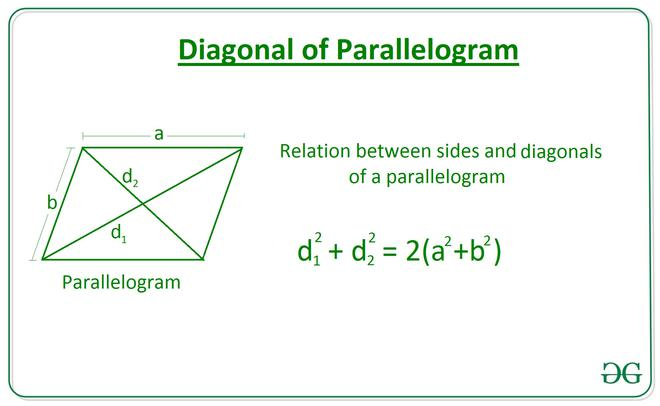Skip to content
Related Articles
Length of a Diagonal of a Parallelogram using the length of Sides and the other Diagonal
• Last Updated : 17 Mar, 2021

Given two integers A and B, denoting the length of a parallelogram and an integer D, denoting the length of a diagonal, the task is to find the length of another diagonal of the parallelogram.

Examples:

Input: A = 10, B = 30, D = 20
Output: 40.0

Input: A = 6, B = 8, D = 10
Output: 10.0

Approach:
The relation between sides and diagonals of a parallelogram length of diagonal is given by the equation:Below is the implementation of the above approach:

## C++

 // C++ Program to implement// the above approach#include using namespace std; // Function to calculate the length// of the diagonal of a parallelogram// using two sides and other diagonalfloat Length_Diagonal(int a, int b, int d){     float diagonal = sqrt(2 * ((a * a) +                               (b * b)) - (d * d));     return diagonal;} // Driver Codeint main(){    int A = 10;    int B = 30;    int D = 20;     // Function Call    float ans = Length_Diagonal(A, B, D);     // Print the final answer    printf("%0.1f", ans);    return 0;} // This code is contributed by Rohit_ranjan

## Java

 // Java Program to implement// the above approachclass GFG{ // Function to calculate the length// of the diagonal of a parallelogram// using two sides and other diagonalstatic float Length_Diagonal(int a, int b, int d){     float diagonal = (float) Math.sqrt(2 * ((a * a) +                                 (b * b)) - (d * d));     return diagonal;} // Driver Codepublic static void main(String[] args){    int A = 10;    int B = 30;    int D = 20;     // Function Call    float ans = Length_Diagonal(A, B, D);     // Print the final answer    System.out.printf("%.1f", ans);}} // This code is contributed by Rajput-Ji

## Python

 # Python Program to implement# the above approach import math # Function to calculate the length# of the diagonal of a parallelogram# using two sides and other diagonaldef Length_Diagonal(a, b, d):     diagonal = math.sqrt(2 * ((a**2) \    + (b**2)) - (d**2))     return diagonal  # Driver CodeA = 10B = 30D = 20 # Function Callans = Length_Diagonal(A, B, D) # Print the final answerprint(round(ans, 2))

## C#

 // C# Program to implement// the above approachusing System;class GFG{ // Function to calculate the length// of the diagonal of a parallelogram// using two sides and other diagonalstatic float Length_Diagonal(int a, int b, int d){     float diagonal = (float) Math.Sqrt(2 * ((a * a) +                                 (b * b)) - (d * d));     return diagonal;} // Driver Codepublic static void Main(String[] args){    int A = 10;    int B = 30;    int D = 20;     // Function Call    float ans = Length_Diagonal(A, B, D);     // Print the readonly answer    Console.Write("{0:F1}", ans);}} // This code is contributed by Rajput-Ji

## Javascript

 
Output:
40.0

Time Complexity: O(1)
Auxiliary Space: O(1)

Attention reader! Don’t stop learning now. Get hold of all the important DSA concepts with the DSA Self Paced Course at a student-friendly price and become industry ready.  To complete your preparation from learning a language to DS Algo and many more,  please refer Complete Interview Preparation Course.

In case you wish to attend live classes with industry experts, please refer Geeks Classes Live

My Personal Notes arrow_drop_up